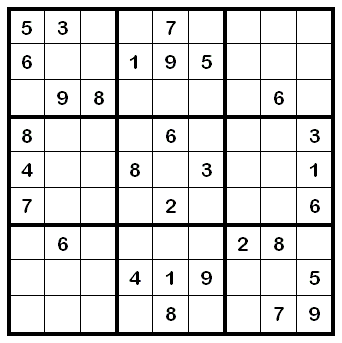Question

# How many different Sudoku's is it possible to make?

Kristján Júlíusson

The number of Sudoku grids on a 9×9 board is 6,670,903,752,021,072,936,960. This number is given in Felgenhauer and Jarvis' article Enumerating possible Sudoku grids. To calculate this number the authors first made several observations on the configurations that needed to be checked, and then used a computer to find those configurations that produced a valid Sudoku grid.

There is no known formula for the number of Sudoku grids on a n×n board. Sudoku grids are a special case of what are termed "Latin squares". These squares/grids, even though having a simple definition, are not easily attacked using the standard techniques of enumeration. Many problems in combinatorics suffer from this problem, however it does not mean that we cannot study properties of these objects.The reason for this is the freedom in producing a valid Sudoku board. In order to make such a board, one must check that each of the 27 sub-configurations (these include all rows, columns and 3*3 blocks) are permutations of {1,2,3,4,5,6,7,8,9}. When producing an exact formula is not possible, mathematicians sometimes resort to giving upper and lower bounds on the number of configurations.

The question concerning the number of different Sudoku's is even harder to answer! In problems of this type it is not immediately clear how to decide when two Sudoku grids are the same. If one rotates a Sudoku grid by 90 degrees then it is easy to see that nothing has changed. If one flips such a grid upside down then, again, it is essentially the same.

However, if one changes each of the numbers 1,2,3,4,5,6,7,8,9 to the numbers 5,8,3,2,6,9,1,4,7 then is it the same? Well, even though this may be less obvious than the other operations, yes of course. From the initial values that one is given, exactly the same reasoning is used to complete the board. There are several other similar operations that one can also do to make a new grid that looks different but is essentially the same.

These operations define what is termed a group in mathematics. Let us call this group G. In order to find the number of different boards, one checks the list of all the configurations on a 9×9 board, looks at the board produced by each of the transformations in the group: if it is already listed then remove it. This will produce a list of all the different Sudoku's on a 9×9 board.

To be more exact, let S be the list of ALL Sudoku's on a 9×9 grid and let L be the empty list. For each grid s in S, if g(s) is in L for some group element g then we do not add s to the list L. Otherwise we add it. Once this has been done for all grids in S we will have a list of different grids L.

The number of these was determined by Russel and Jarvis to be 5,472,730,538.

Picture:

The original question was as follows:
How many different Sudoku's is it possible to make (in the usual size 9x9, as in most newspapers) - and how do you calculate that?

## Um þessa spurningu

### Dagsetning

Published12.3.2008

### Citation

Mark Dukes. „How many different Sudoku's is it possible to make?“. The Icelandic Web of Science 12.3.2008. http://why.is/svar.php?id=7220. (Skoðað 2.12.2023).

### Author

Mark Dukessérfræðingur á stærðfræðistofu við Raunvísindastofnun HÍ